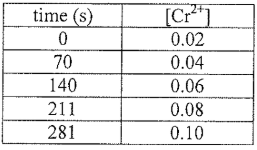# Problem: A student devises an electrochemical cell with the following cell notation:Cr (s) | Cr2+ (aq, 0.02 M) | | Co2+ (aq, 0.9 M) | Co (s)The student monitored the [Cr2+] vs. time. The resultant data is shown in the table below.Unfortunately, at 351 seconds, some solid Pb(NO3)2 spilled into the cathode chamber. the potential rose to 0.796 V. Determine the [Pb2+] present at 351 seconds. Show all work and circle your final answer.

###### FREE Expert Solution

For this problem, we have to calculate the concentration of Pb2+ when the potential rose to 0.796 V

To do so, follow these steps:

Step 1. Determine the Cr2+ produced in 351 s

Step 2. Determine the overall reaction and find n and E°cell

Step 3. Use the Nernst equation to find [Pb2+]

98% (457 ratings)###### Problem Details

A student devises an electrochemical cell with the following cell notation:

Cr (s) | Cr2+ (aq, 0.02 M) | | Co2+ (aq, 0.9 M) | Co (s)

The student monitored the [Cr2+] vs. time. The resultant data is shown in the table below.Unfortunately, at 351 seconds, some solid Pb(NO3)2 spilled into the cathode chamber. the potential rose to 0.796 V. Determine the [Pb2+] present at 351 seconds. Show all work and circle your final answer.